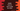# How to sort a kotlin map by value## How to sort a map by value in Kotlin:

Kotlin map is used to hold key-value pairs and using a key, we can access the value that is linked with it. keys in a Kotlin map are unique. We can’t have two same keys. In this post, we will learn how to sort a Kotlin map by its values.

The program will create one map with some key-value pairs and it will sort them by its values.

### Method 1: sort a map by values by converting it to a list:

The idea is to :

• Convert the map to a list.
• sort the list
• Convert the list back to map

Below is the complete program:

``````fun main() {
val givenMap = hashMapOf<String, Int>()
givenMap["one"] = 1
givenMap["two"] = 2
givenMap["three"] = 3
givenMap["four"] = 4
givenMap["five"] = 5
givenMap["six"] = 6

println("Given map :")
givenMap.forEach { (k, v) -> println("\$k => \$v") }

val sortedMap = givenMap.toList().sortedBy { (k, v) -> v }.toMap()

println("Sorted map :")
sortedMap.forEach { (k, v) -> println("\$k => \$v") }
}``````

Here, we have created one hashMap with String as key and Int as the value. givenMap is that variable. We inserted couple of values to this map and printed its key-values using a forEach.

Then we sorted this map by changing it to a list and with the help of sortedBy. The sorted list is converted back to a map using toMap.

### Output:

This program prints the below output:

``````Given map :
six => 6
four => 4
one => 1
two => 2
three => 3
five => 5
Sorted map :
one => 1
two => 2
three => 3
four => 4
five => 5
six => 6``````

### Method 2: Using sortedBy on entries fo the map:

We can use sortedBy method on the entries of the given map. It will sort the pairs based on values if we provide it.value in sortedBy method. These values can be put in a LinkedHashMap :

``````fun main() {
val givenMap = hashMapOf<String, Int>()
givenMap["one"] = 1
givenMap["two"] = 2
givenMap["three"] = 3
givenMap["four"] = 4
givenMap["five"] = 5
givenMap["six"] = 6

println("Given map :")
givenMap.forEach { (k, v) -> println("\$k => \$v") }

givenMap.entries.sortedBy { it.value }.forEach{sortedMap[it.key] = it.value}

println("Sorted map :")
sortedMap.forEach { (k, v) -> println("\$k => \$v") }
}``````

It will print similar result:

``````Given map :
six => 6
four => 4
one => 1
two => 2
three => 3
five => 5
Sorted map :
one => 1
two => 2
three => 3
four => 4
five => 5
six => 6``````

### Method 3: Using entries.sortedWith :

This is almost similar to the above example. The only difference is that we are using sortedWith to sort the items:

``````fun main() {
val givenMap = hashMapOf<String, Int>()
givenMap["one"] = 1
givenMap["two"] = 2
givenMap["three"] = 3
givenMap["four"] = 4
givenMap["five"] = 5
givenMap["six"] = 6

println("Given map :")
givenMap.forEach { (k, v) -> println("\$k => \$v") }

givenMap.entries.sortedWith(java.util.Map.Entry.comparingByValue()).forEach{sortedMap[it.key] = it.value}

println("Sorted map :")
sortedMap.forEach { (k, v) -> println("\$k => \$v") }
}``````

Output:

``````Given map :
six => 6
four => 4
one => 1
two => 2
three => 3
five => 5
Sorted map :
one => 1
two => 2
three => 3
four => 4
five => 5
six => 6``````